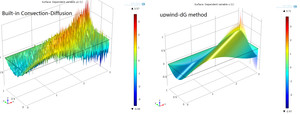# Application ExchangeFilename Size
CDE_uwDG.mph 927 KB
CDE.png 201 KB
up_dG_CDE.png 115 KB

## Convection dominated Convection-Diffusion Equation by upwind discontinuous Galerkin (dG) method

Huashan Sheng (盛华山), Department of Mathematics, Shanghai JiaoTong University (上海交通大学数学系)
Email:

We consider the Convection-Diffusion Equation with very small diffusion coefficient $\mu$:
$-mu\Delta u + \mathbf{\beta}\dot\nabla u =f \mathrm{in}~ \Omega u=g(x,y) \mathrm{on}~ partial\Omega$
First we use the Convection-Diffusion Equation function of The Classical PDE Interfaces in COMSOL 4.4.
Then, we use the WeakForm PDE function and choose shape function type to be discontinuous Largrange.
We compare these two solutions.

Key functions : up() down() nx ny WeakForm PDE
Solving by COMSOL 4.4